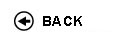Politecnico di Torino
Academic Year 2009/10
17ACFFL, 19ACFFO, 19ACFFP, 19ACFGG
Mathematical analysis I
1st degree and Bachelor-level of the Bologna process in Environmental Engineering - Torino
1st degree and Bachelor-level of the Bologna process in Technology And International Business - Torino/Barcellona
1st degree and Bachelor-level of the Bologna process in Technology And International Business - Torino/Parigi
Espandi...
 Teacher Status SSD Les Ex Lab Tut Years teaching Mazzi LuisaAC MAT/05 70 42 0 0 21
 SSD CFU Activities Area context MAT/05 10 A - Di base Matematica, informatica e statistica
 Objectives of the course This course is a link between high school and university. Our students come from different types of high schools, so we aim to homogenize the basic knowledge of students within a short time (almost two weeks). The basics, like inequalities, elementary graphs, trigonometry, are studied in depth and under a more general perspective. The aim of this course is to train students at the university system, and to teach them the basis of differential and integral calculus for variable functions, with applications on first order ordinary differential equations and on second order linear equations. Complex numbers are studied as well and they are applied to represent the solutions of second order ordinary differential equations. To representa ordinary differential equations it is necessary to know the concept of a partial derivative and the fondamental concepts on functions continuity of many variables and on partial derivatives. Many applications of differential and integral calculus are shown. Expected skills Skills to understand a logic chain; comprehension of basic properties of differential and integral calculus for a variable function. Acquisition of good skills in calculation. Skills in reading a technical text. Syllabus Rudiments of sets. Numerical sets. Real functions of a real variable: characteristics and elementary funtions. Limits: definition and basic limits. Continuity and global properties of continuous functions in an interval. Derivatives and differential: derivatives of elementary functions and derivative rules. Theorems of differential calculus and applications: critical points; global functions properties in an interval. Convex functions. De l'Hospital's theorems. Local functions comparison: infinity, infinitesimals and their classification. Taylor's formula and its applications. Primitives. Integral calculus: integral of a scale function. Integral of a limited function. Basic properties of an integral. Integral function and foundamental theorem of integralc alculus. Indefinite integral and foundamental formula of ain integral calculus. Integration methods and rules. Improper integrals. Basics of first and second order differential equations. Check availability at the library Programma provvisorio per l'A.A.2005/06© Politecnico di Torino
Corso Duca degli Abruzzi, 24 - 10129 Torino, ITALY